• +91 9971497814
• info@interviewmaterial.com

# Chapter 9 Areas of Parallelograms and Triangles Ex- 9.2 Interview Questions Answers

### Related Subjects

Question 1 : In figure, ABCD is a parallelogram, AE DC and CF AD. If AB = 16 cm, AE = 8 cm and CF = 10 cm, find AD.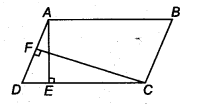Answer 1 : We know that,
Area of parallelogram = Base x Altitude
Given, AE = 8 cm CF = 10 cm and AB = 16cm
ar (parallelogramABCD) =DC x AE
= 16 x 8 cm2 (
AE = 8 cm)…(i)
and ar (parallelogram ABCD) = AD x CF – AD x 10 (
CF = 10 cm)
From Eq. (i), we have,
16 x 8 = AD x 10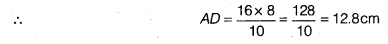Question 2 :

If E, F, G and H are respectively the mid-points of the sides of aparallelogram ABCD, show that ar (EFGH) = 1/2 ar(ABCD).

Answer 2 :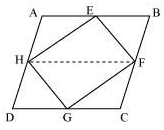Given,

E, F, G and H are themid-points of the sides of a parallelogram ABCD respectively.

To Prove,

ar (EFGH) = ½ ar(ABCD)

Construction,

H and F are joined.

Proof,

Also,

AH || BF and and DH ||CF

AH = BF and DH = CF(H and F are mid points)

, ABFH and HFCD areparallelograms.

Now,

We know that, ΔEFH andparallelogram ABFH, both lie on the same FH the common base and in-between thesame parallel lines AB and HF.

area of EFH = ½ areaof ABFH — (i)

And, area of GHF = ½area of HFCD — (ii)

area of ΔEFH +area of ΔGHF = ½ area of ABFH + ½ area of HFCD

area of EFGH = areaof ABFH

ar (EFGH) = ½ar(ABCD)

Question 3 :

Pand Q are any two points lying on the sides DC and AD respectively of aparallelogram ABCD. Show that ar (APB) = ar (BQC).

Answer 3 :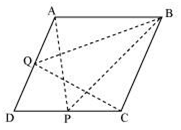Itcan be observed that ΔBQC and parallelogram ABCD lie on the same base BC andthese are between the same parallel lines AD and BC.

Area (ΔBQC) =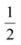Area (ABCD) … (1)

Similarly,ΔAPB and parallelogram ABCD lie on the same base AB and between the sameparallel lines AB and DC.

Area (ΔAPB) =Area (ABCD) … (2)

Fromequation (1) and (2), we obtain

Area(ΔBQC) = Area (ΔAPB)

Question 4 :

Inthe given figure, P is a point in the interior of a parallelogram ABCD. Showthat

(i)ar (APB) + ar (PCD) =ar (ABCD)

(ii)ar (APD) + ar (PBC) = ar (APB) + ar (PCD)

[Hint:Through. P, draw a line parallel to AB]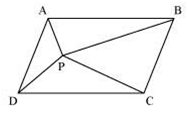Answer 4 :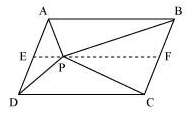Let us draw a line segmentMN, passing through point P and parallel to line segment AD.

In parallelogram ABCD,

MN || AD (By construction)… (6)

ABCD is a parallelogram.

AB || DC (Opposite sides of a parallelogram)

AM || DN … (7)

From equations (6) and(7), we obtain

MN || AD and AM || DN

It can be observed thatΔAPD and parallelogram AMND are lying on the same base AD and between the sameparallel lines AD and MN.

Area (ΔAPD) =Area (AMND) … (8)

Similarly, for ΔPCB andparallelogram MNCB,

Area(ΔPCB) =Area (MNCB) … (9)
Addingequations (8) and (9), we obtain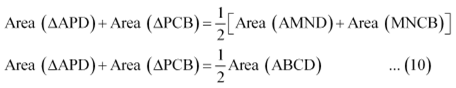On comparing equations (5)and (10), we obtain

Area (ΔAPD) + Area (ΔPBC)= Area (ΔAPB) + Area (ΔPCD)

Question 5 :

In the given figure, PQRSand ABRS are parallelograms and X is any point on side BR. Show that

(i) ar (PQRS) = ar (ABRS)

(ii)ar (AXS) =ar (PQRS)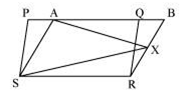(i) It can be observedthat parallelogram PQRS and ABRS lie on the same base SR

and also, these lie inbetween the same parallel lines SR and PB.

Area (PQRS) = Area (ABRS) … (1)

(ii) Consider ΔAXS andparallelogram ABRS.

As these lie on the samebase and are between the same parallel lines AS and BR,

Area (ΔAXS) =Area (ABRS) … (2)

From equations (1) and(2), we obtain

Area(ΔAXS) =Area (PQRS)

Question 6 :

Afarmer was having a field in the form of a parallelogram PQRS. She took anypoint A on RS and joined it to points P and Q. In how many parts the field isdivided? What are the shapes of these parts? The farmer wants to sow wheat andpulses in equal portions of the field separately. How should she do it?

Answer 6 :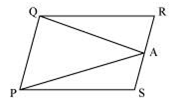From the figure, it can beobserved that point A divides the field into three parts. These parts aretriangular in shape − ΔPSA, ΔPAQ, and ΔQRA

Areaof ΔPSA + Area of ΔPAQ + Area of ΔQRA = Area of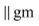PQRS … (1)

We know that if aparallelogram and a triangle are on the same base and between the sameparallels, then the area of the triangle is half the area of the parallelogram.

Area (ΔPAQ) =Area (PQRS) … (2)

From equations (1) and(2), we obtain

Area(ΔPSA) + Area (ΔQRA) =Area (PQRS) … (3)

Clearly,it can be observed that the farmer must sow wheat in triangular part PAQ andpulses in other two triangular parts PSA and QRA or wheat in triangular partsPSA and QRA and pulses in triangular parts PAQ.

Todays Deals### Chapter 9 Areas of Parallelograms and Triangles Ex- 9.2 Contributorskrishan

Name:
Email:

# Latest News# 9000 interview questions in different categories